How Cheenta works to ensure student success?
Explore the Back-Story

# Test of Mathematics Solution Subjective 69 - Coefficients of PolynomialThis is a Test of Mathematics Solution Subjective 69 (from ISI Entrance). The book, Test of Mathematics at 10+2 Level is Published by East West Press. This problem book is indispensable for the preparation of I.S.I. B.Stat and B.Math Entrance.

Also visit: I.S.I. & C.M.I. Entrance Course of Cheenta

## Problem

Suppose that the three equations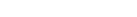,, and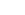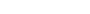all have only positive roots. Show that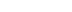.

## Solution

If possible let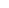,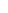,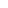are not all equal,,all have only positive roots. So all of,,cannot be its same sign as discriminant > 0 for three equations (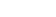,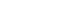,). Without loss of generality we can assume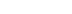oras the equations are cyclic. Now we know,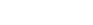,,. Now there 2 possibilities either b, c both are positive or one of b, c is positive. If b, c both are positive then,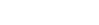[ not possible ]. If one of b, c is positive then,[ not possible ] So a, b, c have to be equal.This is a Test of Mathematics Solution Subjective 69 (from ISI Entrance). The book, Test of Mathematics at 10+2 Level is Published by East West Press. This problem book is indispensable for the preparation of I.S.I. B.Stat and B.Math Entrance.

Also visit: I.S.I. & C.M.I. Entrance Course of Cheenta

## Problem

Suppose that the three equations,, andall have only positive roots. Show that.

## Solution

If possible let,,are not all equal,,all have only positive roots. So all of,,cannot be its same sign as discriminant > 0 for three equations (,,). Without loss of generality we can assumeoras the equations are cyclic. Now we know,,,. Now there 2 possibilities either b, c both are positive or one of b, c is positive. If b, c both are positive then,[ not possible ]. If one of b, c is positive then,[ not possible ] So a, b, c have to be equal.

### Knowledge Partner